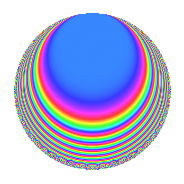# Properties

 Label 672.2.i.eLevel 672 Weight 2 Character orbit 672.i Analytic conductor 5.366 Analytic rank 0 Dimension 8 CM disc. -56 Inner twists 8

# Related objects

## Newspace parameters

 Level: $$N$$ = $$672 = 2^{5} \cdot 3 \cdot 7$$ Weight: $$k$$ = $$2$$ Character orbit: $$[\chi]$$ = 672.i (of order $$2$$ and degree $$1$$)

## Newform invariants

 Self dual: No Analytic conductor: $$5.36594701583$$ Analytic rank: $$0$$ Dimension: $$8$$ Coefficient field: 8.0.10070523904.11 Coefficient ring: $$\Z[a_1, \ldots, a_{7}]$$ Coefficient ring index: $$2^{3}\cdot 3$$ Sato-Tate group: $\mathrm{U}(1)[D_{2}]$

## $q$-expansion

Coefficients of the $$q$$-expansion are expressed in terms of a basis $$1,\beta_1,\ldots,\beta_{7}$$ for the coefficient ring described below. We also show the integral $$q$$-expansion of the trace form.

 $$f(q)$$ $$=$$ $$q + \beta_{1} q^{3} + ( \beta_{6} - \beta_{7} ) q^{5} + \beta_{3} q^{7} + ( \beta_{2} + \beta_{3} ) q^{9} +O(q^{10})$$ $$q + \beta_{1} q^{3} + ( \beta_{6} - \beta_{7} ) q^{5} + \beta_{3} q^{7} + ( \beta_{2} + \beta_{3} ) q^{9} + ( -2 \beta_{1} - \beta_{6} - \beta_{7} ) q^{13} + ( 1 + 2 \beta_{2} - \beta_{3} + \beta_{4} ) q^{15} + ( -\beta_{1} + \beta_{5} - \beta_{6} - \beta_{7} ) q^{19} + ( \beta_{5} + \beta_{6} ) q^{21} -2 \beta_{4} q^{23} + ( -5 - 2 \beta_{3} ) q^{25} + ( \beta_{5} + \beta_{6} - \beta_{7} ) q^{27} + ( 3 \beta_{1} - \beta_{5} + \beta_{6} - 3 \beta_{7} ) q^{35} + ( -5 - 4 \beta_{2} - \beta_{3} + \beta_{4} ) q^{39} + ( 2 \beta_{1} - 2 \beta_{5} + \beta_{6} - 3 \beta_{7} ) q^{45} + 7 q^{49} + ( -1 - 5 \beta_{2} + \beta_{3} + 2 \beta_{4} ) q^{57} + ( -3 \beta_{1} + \beta_{5} + 3 \beta_{6} - \beta_{7} ) q^{59} + ( 4 \beta_{1} + 2 \beta_{5} + \beta_{6} + \beta_{7} ) q^{61} + ( 7 + \beta_{4} ) q^{63} + ( -2 \beta_{2} - 4 \beta_{4} ) q^{65} + ( -2 \beta_{1} + 2 \beta_{5} - 4 \beta_{6} + 2 \beta_{7} ) q^{69} -4 \beta_{2} q^{71} + ( -5 \beta_{1} - 2 \beta_{5} - 2 \beta_{6} ) q^{75} + 2 \beta_{3} q^{79} + ( 5 + 2 \beta_{4} ) q^{81} + ( -3 \beta_{1} + \beta_{5} - 3 \beta_{6} + 5 \beta_{7} ) q^{83} + ( -\beta_{1} - 3 \beta_{5} + \beta_{6} + \beta_{7} ) q^{91} + ( 8 \beta_{2} - 2 \beta_{4} ) q^{95} +O(q^{100})$$ $$\operatorname{Tr}(f)(q)$$ $$=$$ $$8q + O(q^{10})$$ $$8q + 8q^{15} - 40q^{25} - 40q^{39} + 56q^{49} - 8q^{57} + 56q^{63} + 40q^{81} + O(q^{100})$$

Basis of coefficient ring in terms of a root $$\nu$$ of $$x^{8} - 10 x^{4} + 81$$:

 $$\beta_{0}$$ $$=$$ $$1$$ $$\beta_{1}$$ $$=$$ $$\nu$$ $$\beta_{2}$$ $$=$$ $$($$$$\nu^{6} - \nu^{2}$$$$)/18$$ $$\beta_{3}$$ $$=$$ $$($$$$-\nu^{6} + 19 \nu^{2}$$$$)/18$$ $$\beta_{4}$$ $$=$$ $$($$$$\nu^{4} - 5$$$$)/2$$ $$\beta_{5}$$ $$=$$ $$($$$$-\nu^{7} - 9 \nu^{5} + 37 \nu^{3} + 63 \nu$$$$)/54$$ $$\beta_{6}$$ $$=$$ $$($$$$-2 \nu^{7} + 9 \nu^{5} + 20 \nu^{3} - 63 \nu$$$$)/54$$ $$\beta_{7}$$ $$=$$ $$($$$$-\nu^{7} + \nu^{3}$$$$)/18$$
 $$1$$ $$=$$ $$\beta_0$$ $$\nu$$ $$=$$ $$\beta_{1}$$ $$\nu^{2}$$ $$=$$ $$\beta_{3} + \beta_{2}$$ $$\nu^{3}$$ $$=$$ $$-\beta_{7} + \beta_{6} + \beta_{5}$$ $$\nu^{4}$$ $$=$$ $$2 \beta_{4} + 5$$ $$\nu^{5}$$ $$=$$ $$-2 \beta_{7} + 4 \beta_{6} - 2 \beta_{5} + 7 \beta_{1}$$ $$\nu^{6}$$ $$=$$ $$\beta_{3} + 19 \beta_{2}$$ $$\nu^{7}$$ $$=$$ $$-19 \beta_{7} + \beta_{6} + \beta_{5}$$

## Character Values

We give the values of $$\chi$$ on generators for $$\left(\mathbb{Z}/672\mathbb{Z}\right)^\times$$.

 $$n$$ $$127$$ $$421$$ $$449$$ $$577$$ $$\chi(n)$$ $$1$$ $$-1$$ $$-1$$ $$-1$$

## Embeddings

For each embedding $$\iota_m$$ of the coefficient field, the values $$\iota_m(a_n)$$ are shown below.

For more information on an embedded modular form you can click on its label.

Label $$\iota_m(\nu)$$ $$a_{2}$$ $$a_{3}$$ $$a_{4}$$ $$a_{5}$$ $$a_{6}$$ $$a_{7}$$ $$a_{8}$$ $$a_{9}$$ $$a_{10}$$
209.1
 −1.68014 − 0.420861i −1.68014 + 0.420861i −0.420861 − 1.68014i −0.420861 + 1.68014i 0.420861 − 1.68014i 0.420861 + 1.68014i 1.68014 − 0.420861i 1.68014 + 0.420861i
0 −1.68014 0.420861i 0 3.91044i 0 2.64575 0 2.64575 + 1.41421i 0
209.2 0 −1.68014 + 0.420861i 0 3.91044i 0 2.64575 0 2.64575 1.41421i 0
209.3 0 −0.420861 1.68014i 0 2.16991i 0 −2.64575 0 −2.64575 + 1.41421i 0
209.4 0 −0.420861 + 1.68014i 0 2.16991i 0 −2.64575 0 −2.64575 1.41421i 0
209.5 0 0.420861 1.68014i 0 2.16991i 0 −2.64575 0 −2.64575 1.41421i 0
209.6 0 0.420861 + 1.68014i 0 2.16991i 0 −2.64575 0 −2.64575 + 1.41421i 0
209.7 0 1.68014 0.420861i 0 3.91044i 0 2.64575 0 2.64575 1.41421i 0
209.8 0 1.68014 + 0.420861i 0 3.91044i 0 2.64575 0 2.64575 + 1.41421i 0
 $$n$$: e.g. 2-40 or 990-1000 Embeddings: e.g. 1-3 or 209.8 Significant digits: Format: Complex embeddings Normalized embeddings Satake parameters Satake angles

## Inner twists

Char. orbit Parity Mult. Self Twist Proved
1.a Even 1 trivial yes
56.h Odd 1 CM by $$\Q(\sqrt{-14})$$ yes
3.b Odd 1 yes
7.b Odd 1 yes
8.b Even 1 yes
21.c Even 1 yes
24.h Odd 1 yes
168.i Even 1 yes

## Hecke kernels

This newform can be constructed as the intersection of the kernels of the following linear operators acting on $$S_{2}^{\mathrm{new}}(672, [\chi])$$:

 $$T_{5}^{4} + 20 T_{5}^{2} + 72$$ $$T_{11}$$ $$T_{13}^{4} - 52 T_{13}^{2} + 648$$